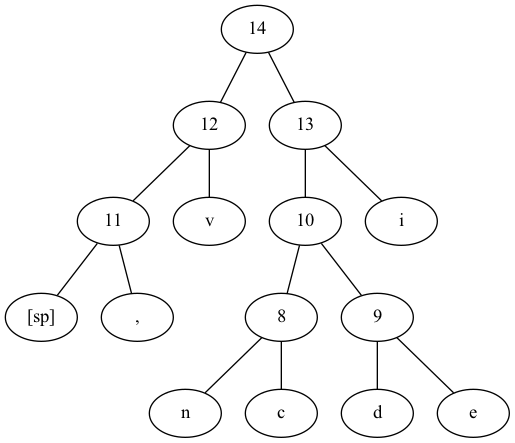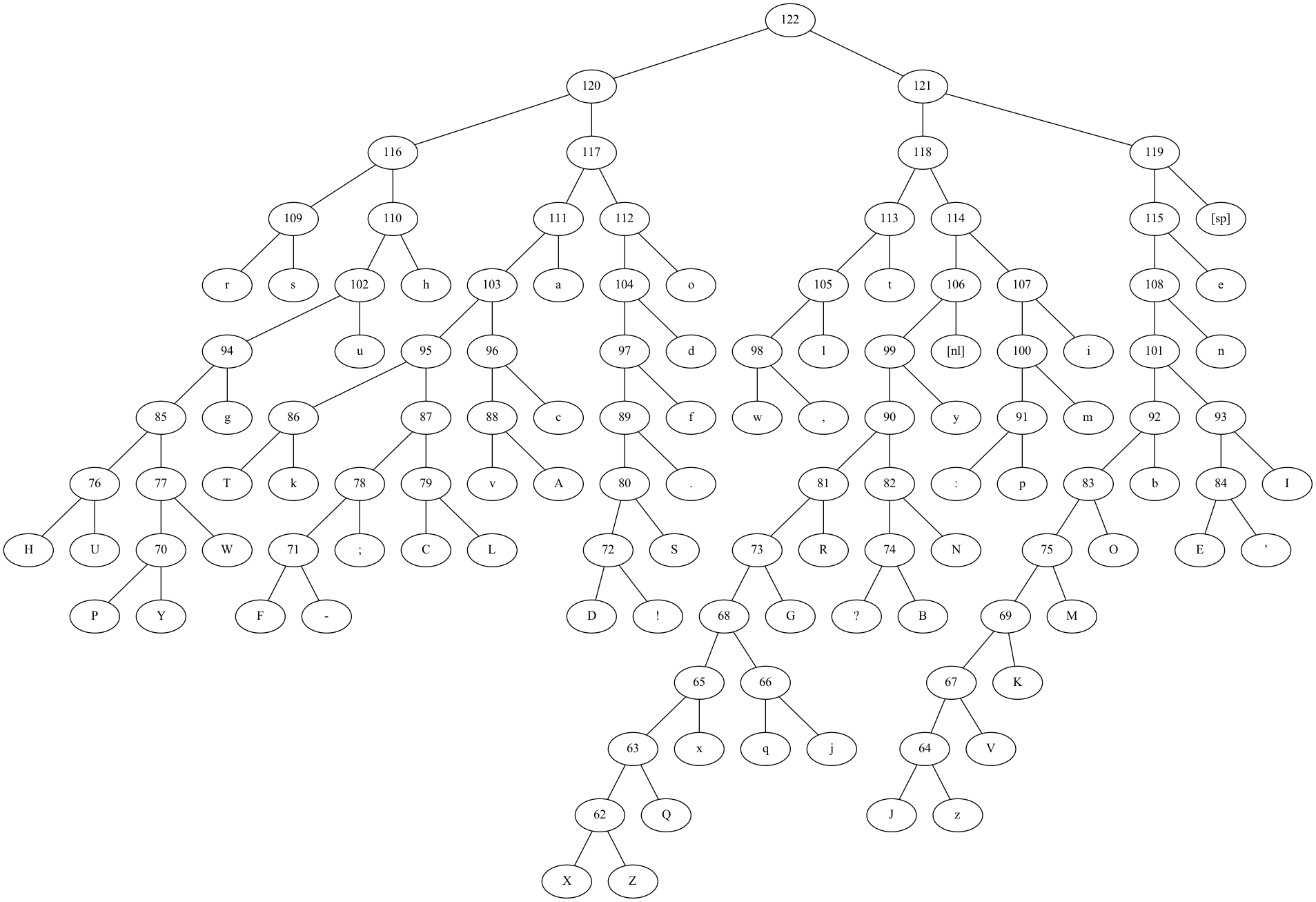# kleemans.ch

## Huffman coding

July 4, 2022

Compression is an interesting task. How can we compress data - like text - so that it takes up less space, with the ability to fully restore the original data? (By the way, how to do that in the best way possible for a certain dataset is still an ongoing task, have a look at the Hutter Prize!)

A pretty good and widely used way is Huffman coding, which I'll be implementing in this post.

### Assigning codes to characters

One important concept is to assign "codes" to the characters of a source text, and then optimize which character gets which code. For example, ASCII itself is already a character to code assignment. If we have a source text of `veni, vidi, vici`, the ASCII codes are as follows:

``````v = 0111 0110
e = 0110 0101
n = 0110 1110
i = 0110 1001
, = 0010 1100
_ = 0010 0000
v = 0111 0110
i = 0110 1001
d = 0110 0100
i = 0110 1001
, = 0010 1100
_ = 0010 0000
v = 0111 0110
i = 0110 1001
c = 0110 0011
i = 0110 1001``````

Every character takes up 8 bits, so the total message `veni, vidi, vici` takes up 16 * 8 = 128 bits.

But what if we use shorter codes? As we only have 8 different characters, we can just assign numbers from 1 to 8:

``````e = 000
c = 001
d = 010
i = 011
n = 100
v = 101
, = 110
_ = 111``````

Now we only use 3 bits per character, totalling in 16 * 3 = 48 bits. But is it possible to improve this?

### Huffman coding

The message above has the characters `v` and `i` multiple times. So maybe can we just assign shorter codes to those, but longer codes to characters which appear only once (like `c` and `d`)?

Also, the catch is that if we use a short code like `10` for a character, we can't use that sequence at the start of any other code, because then we wouldn't know how to decompress it if we encounter it in a compressed text.

That is exactly the motivation behind optimizing compression, and Huffman came up with a "theoretically optimal" method (for this class of methods).

The basic algorithm is pretty simple and goes like this:

1. First, add a node for each character to a queue, with the weight as the frequency of appearance.
2. While there is still more than one node in the queue:
• Remove the two lowest-weighted nodes and create a new one with the combined weight, setting the previous nodes as children
• Add the new node to the queue and re-sort the queue
3. The last node is the root note.

Or, in code:

``````# Node class
class Node(object):
def __init__(self, idx, symbol, weight, child1=None, child2=None):
self.idx = idx
self.symbol = symbol
self.weight = weight
self.child1 = child1
self.child2 = child2
self.bits = ''

# Initialize nodes - data holds the message, chars contains all the different characters
nodes = []
idx = 0
for c in chars:
n = Node(str(idx), c, data.count(c))
idx += 1
nodes.append(n)
nodes.sort(key=lambda x: x.weight, reverse=True)

# Build tree
while len(nodes) > 1:
nodes.sort(key=lambda x: x.weight, reverse=True)
# Build new node
c = [nodes.pop(), nodes.pop()]
n = Node(str(idx), str(idx), c.weight + c.weight, c, c)
idx += 1
nodes.append(n)``````

See here for a more in-depth explanation:

If we apply this to our message, we get the following encoding:

``````idx     char    count   code
2       "i"     5       11
7       "v"     3       00
3       ","     2       011
6       "[sp]"  2       010
0       "e"     1       1001
1       "d"     1       1000
4       "c"     1       1011
5       "n"     1       1010``````

The tree looks like this:With this, we can compress our original message to `00100110111101101000111010110110100011100011` - only using 44 bits!

### Applying it to more text

How does this work for bigger texts? If we take the complete works of William Shakespeare, the output is as follows:

``````id      symbol  count   code
2       "[sp]"  169858  110
11      "e"     94611   1110
3       "t"     67009   1000
30      "o"     65798   0111
23      "a"     55507   0100
52      "h"     51310   0010
61      "s"     49696   0001
56      "r"     48889   0000
27      "n"     48529   11111
15      "i"     45537   10111
12      "[nl]"  40004   10100
33      "l"     33340   10010
40      "d"     31358   01100
48      "u"     26584   00110
29      "m"     22243   101101
42      "y"     20448   101011
5       ","     19843   100111
36      "w"     17585   100110
28      "f"     15770   011010
39      "c"     15622   010111
7       "g"     13356   001111
25      "I"     11832   1111010
6       "b"     11321   1111000
41      "p"     10808   1011001
13      ":"     10316   1011000
16      "."     7885    0110110
10      "A"     7819    0101101
44      "v"     7793    0101100
49      "k"     7088    0101001
24      "T"     7015    0101000
38      "'"     6187    11110111
31      "E"     6041    11110110
43      "O"     5481    11110010
37      "N"     5079    10101010
26      "R"     4869    10101001
50      "S"     4523    01101111
57      "L"     3876    01010111
4       "C"     3818    01010110
45      ";"     3628    01010100
46      "W"     3530    00111011
9       "U"     3313    00111001
34      "H"     3068    00111000
22      "M"     2840    111100110
21      "B"     2761    101010111
60      "?"     2462    101010110
51      "G"     2399    101010001
17      "!"     2172    011011101
47      "D"     2089    011011100
1       "-"     1897    010101011
18      "F"     1797    010101010
0       "Y"     1718    001110101
58      "P"     1641    001110100
59      "K"     1584    1111001111
14      "V"     798     11110011101
8       "j"     628     10101000011
35      "q"     609     10101000010
32      "x"     529     10101000000
55      "z"     356     111100111001
19      "J"     320     111100111000
53      "Q"     231     101010000010
54      "Z"     198     1010100000111
20      "X"     112     1010100000110``````

Or, visualized, it looks like this:The compression ratio is as follows:

• Original file `shakespeare.txt`: 1'115'328 bytes
• Compressed file `shakespeare.bin`: 671'524 bytes

So the compressed data is only 60.2% of the original data!

By the way, to decompress it, we can just run read through the bits and if we found a matching sequence, write it to an output:

``````_bytes = numpy.fromfile('compressed.bin', dtype="uint8")
bits = numpy.unpackbits(_bytes)
with open('decompressed.txt', 'w') as write_file:
buffer = ''
for bit in bits:
buffer += str(bit)
if buffer in nodes:
write_file.write(nodes.symbol)
buffer = ''``````

For the graphs, pydot was used. All code can be found here.